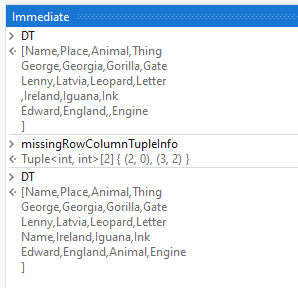# How to replace empty cells with its corresponding column name

So i have a scenario where i want to replace each empty data in a data table to its coulmn name

currently im trying to loop foreach = dt.Columns

inside here im having a for each row in data table

but now im blank for what to be done next

and also a linq query will be more helpfull

For One Particular Speficed Column You can use this

Reverse your For Each Row and For Each. Put the For Each in the For Each Row in Datatable.

Inside the For Each, put an If with String.IsNullOrEmpty(CurrentRow(currentItem.ColumnName).ToString) as your condition. Inside the If, put an Assign with CurrentRow(currentItem.ColumnName) = currentItem.ColumnName

Make sure the TypeArgument of your For Each is System.Data.DataColumn.

@sagar.raval

Try this

`dataTable.AsEnumerable().SelectMany(function(x) x.ItemArray.Select(function(v, i) => new { Row = c, Value = v, Index = i })) .Where(function(x) string.IsNullOrEmpty(item.Value.ToString())) .ToList() .ForEach(function(x) = x.Row(x.Index) = dataTable.Columns(x.Index).ColumnName)`

Cheers

Hi @sagar.raval ,

We could also check with the below Steps :

1. Firstly, Get the row and column indices of the Missing Cell values. We can use the below Expression :
``````missingRowColumnTupleInfo = (From x In DT.AsEnumerable
From y In x.ItemArray
Where String.IsNullOrWhiteSpace(y.ToString)
Select Tuple.Create(DT.Rows.IndexOf(x),x.ItemArray.ToList.IndexOf(y))).ToArray
``````

Here, `missingRowColumnTupleInfo` is a variable of Type `Array of Tuple(Of Integer,Integer)`

1. Next, we can loop the create Tuple Array which will consists of row and Column Indices of the Missing cell values and update the Datatable on those indices accordingly :
``````DT.Rows(currentTuple.Item1)(currentTuple.Item2) = DT.Columns(currentTuple.Item2).ColumnName
``````

1. At the end, we can write the Updated datatable back to the Excel sheet using `Write Range` activity.

Debug Visuals :